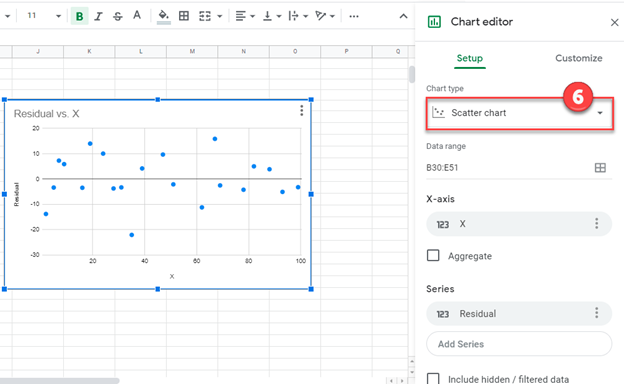# Calculate & Plot Residuals – Excel & Google Sheets

This tutorial will demonstrate how to calculate and plot residuals in Excel and Google Sheets.

## Calculate & Plot Residuals – Excel

We’ll start with this dataset containing values for the X and Y Axis.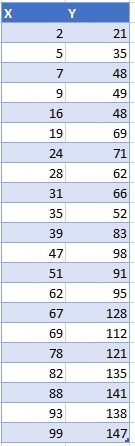### Creating a Scatterplot

2. Click Insert
3. Select Scatterplot
4. Select the first Scatterplot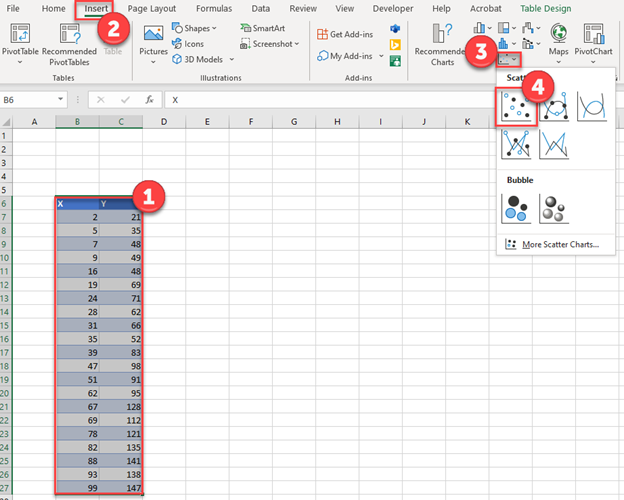The Scatterplot should look similar to the one below.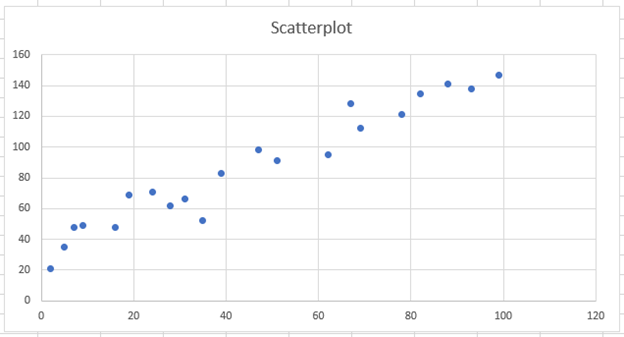### Trendline

2. Select the Arrow next to Trendline
3. Click More Options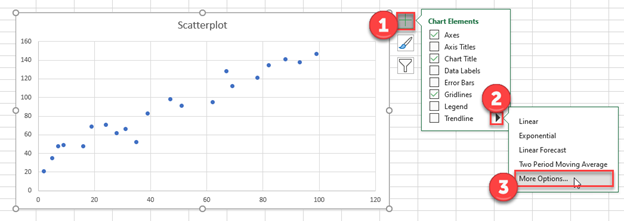4. Select Linear Trendline

5. Check Display Equation on Chart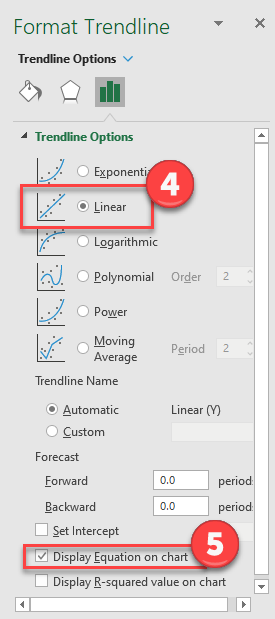The trendline looks similar to the one below, that also shows the equation.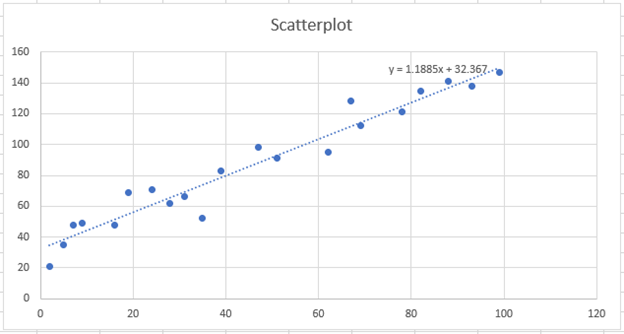### Copy and Paste Equation

1. Highlight the equation and copy (CTRL + C)
2. Paste the equation on the worksheet (CTRL + V)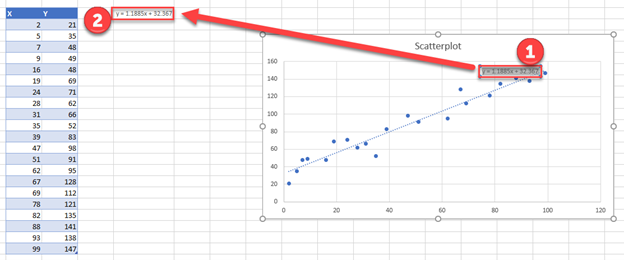### Calculate Values

Using the equation from the previous step, create a formula: replacing x with the X Value in the table. Drag this formula down for all of the values.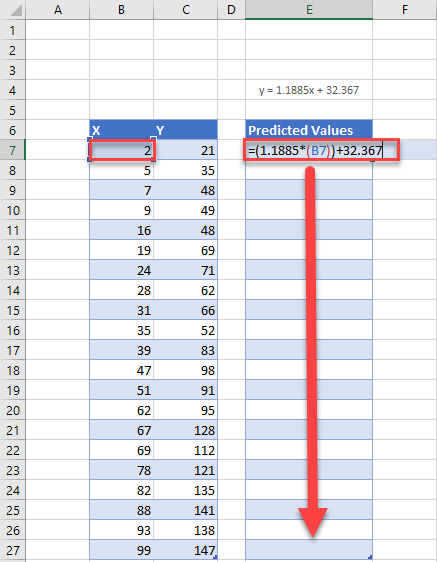### Easy Excel Automation### Table with Predicted Values

This formula calculates the predicted Y-axis values.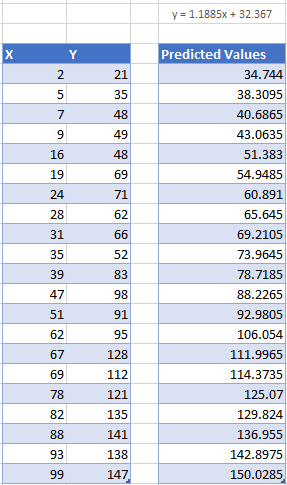### Find Residuals

Find the residuals by using the formula = Y Value – Predicted Values. Repeat this for all Values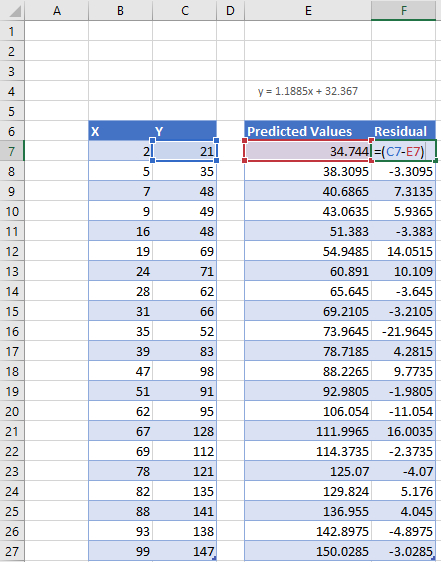### Creating Residual Plot

1. Highlight the X Values
2. Hold down CTRL and highlight Residuals
3. Click Insert
4. Select Scatter
5. Click the first Scatterplot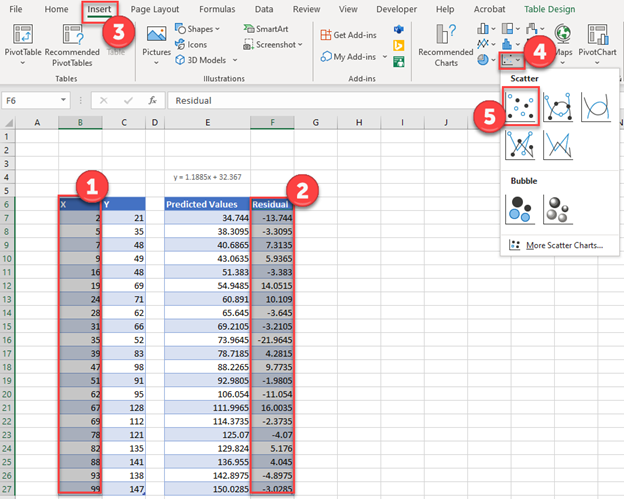### Final Residual Plot

This is the final residual plot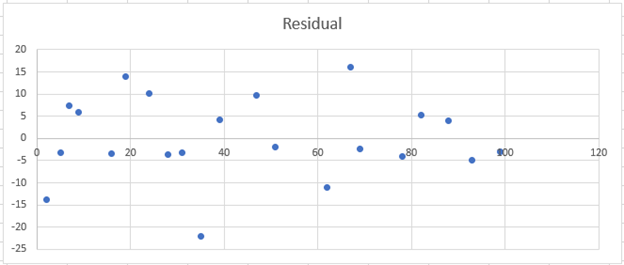## Calculate & Plot Residuals – Google Sheets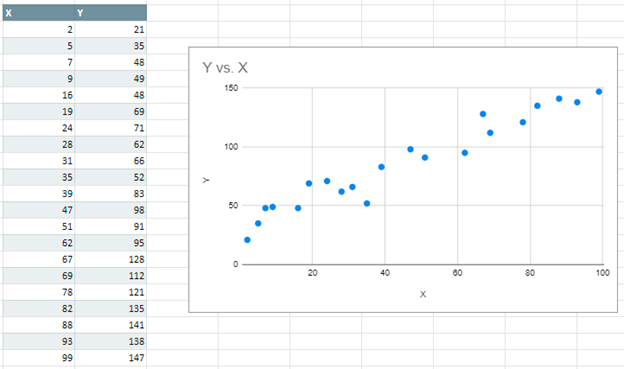### Creating a Trendline

1. Double Click Graph
2. Select Customize in Chart Editor
3. Click Series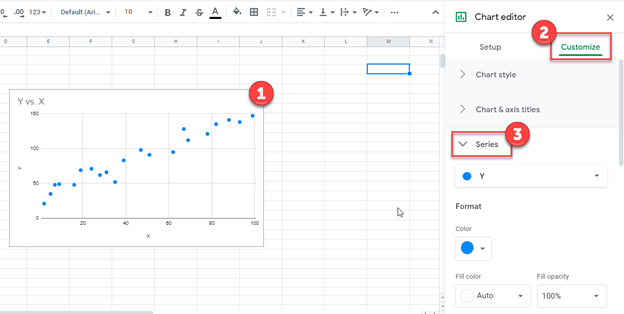4. Check Trendline

5. Select Use Equation under Label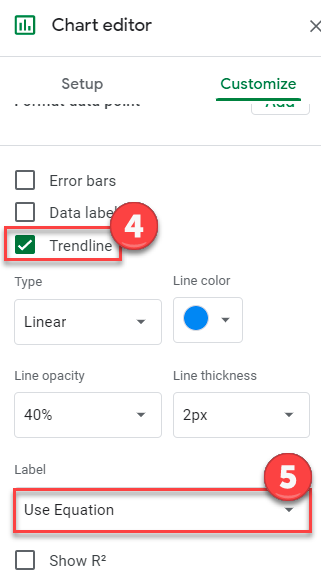### Predicted Values

Similar to the Excel example above, plug in the X Value in the equation that was given as shown below. Do this for all of the Values. This gives us the predicted Y-Axis values.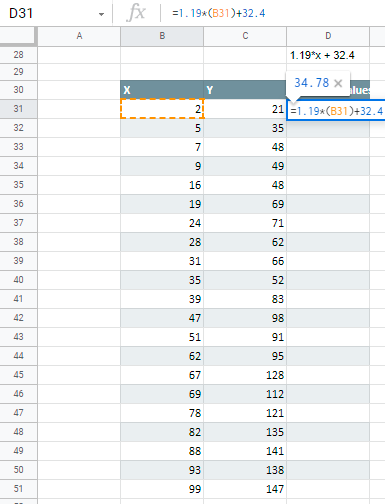### Residual Values

Similar to Excel, you’ll repeat the same formula where =Y Value – Predicted Value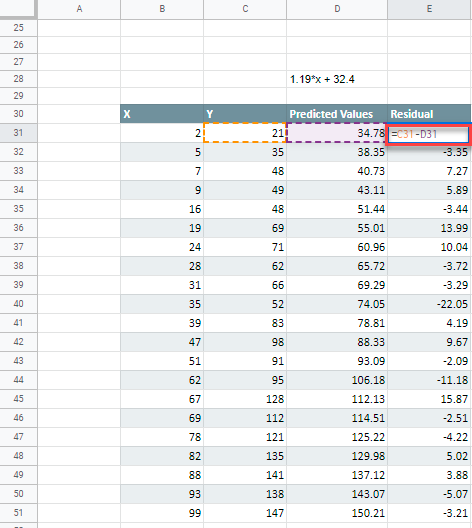### Creating Residual Plot

1. Highlight Y Value and Predicted Values and Right Click
2. Select Hide Column C-D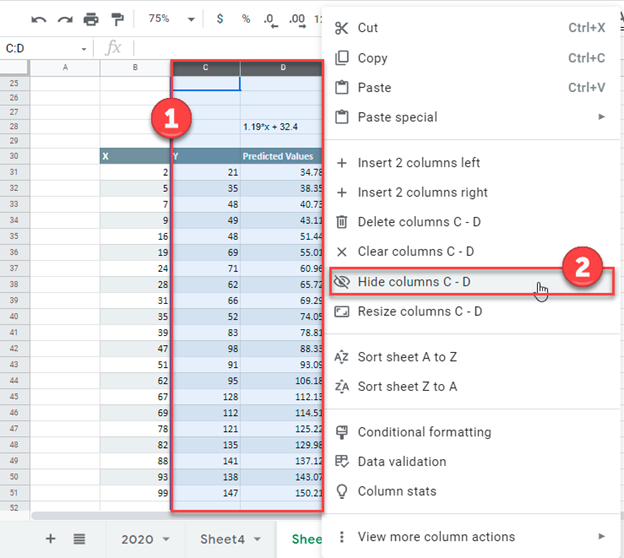3. Highlight X and Residual Values

4. Select Insert

5. Click Chart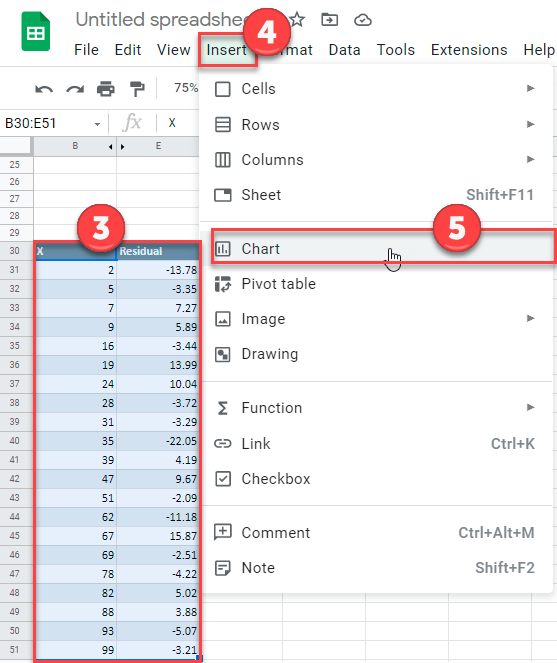6. Change Chart Type to Scatter Chart